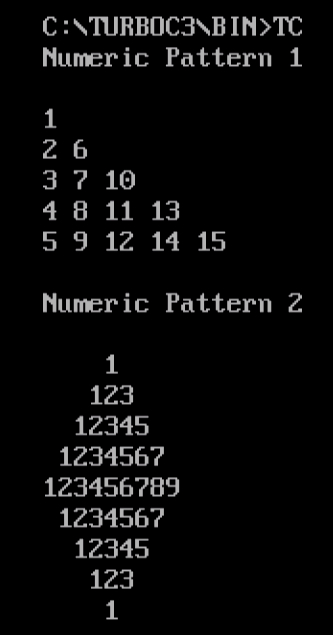# Program to print number pattern in C

## Program Description

A numerical pattern is a sequence of numbers that have been created based on a rule called a pattern rule. Pattern rules can use one or more mathematical operations to describe the relationship between consecutive numbers in the sequence.

Examples for Patterns

Pattern 1

1
2 6
3 7 10
4 8 11 13
5 9 12 14 15

Pattern 2

        1
1 2 3
1 2 3 4 5
1 2 3 4 5 6 7
1 2 3 4 5 6 7 8 9
1 2 3 4 5 6 7
1 2 3 4 5
1 2 3
1

## Algorithm

Pattern 1:
i stands for rows and j stands for columns.
5 stands for making pattern for 5 Rows and Columns
Loop for each Row (i)
K is initialized to i
Loop for each Column (j)
Do the Pattern for the current Column (j)
Display the Value of K
Reinitialize the Value of K = k + 5 - j
Pattern 2:
First Row: Display 1
Second Row: Display 1,2,3
Third Row: Display 1,2,3,4,5
Fourth Row: Display 1,2,3,4,5,6,7
Fifth Row: Display 1,2,3,4,5,6,7,8,9
Display the same contents from 4th Row till First Row below the fifth Row.

## Example

/* Program to print Numeric Pattern */
#include<stdio.h>
int main(){
int i,j,k;
printf("Numeric Pattern 1");
printf("");
printf("");
for(i=1;i<=5;i++){
k = i;
for(j=1;j<=i;j++){
printf("%d ", k);
k += 5-j;
}
printf("");
}
printf("");
printf("Numeric Pattern 2");
printf("");
printf("");
for(i = 1;i<=5;i++){
for(j = i;j<5;j++){
printf(" ");
}
for(k = 1;k<(i*2);k++){
printf("%d",k);
}
printf("");
}
for(i = 4;i>=1;i--){
for(j = 5;j>i;j--){
printf(" ");
}
for(k = 1;k<(i*2);k++){
printf("%d",k);
}
printf("");
}
getch();
return 0;
}

## Output